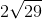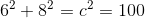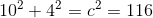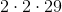Scan QR code or get instant email to install app

Question:

# A rectangular prism has a width of 6 meters, a height of 4 meters, and a length of 8 meters. What is the distance from the bottom right corner of the 6 by 4 front side to the top left corner of the 6 by 4 back side?

A 1212explanation

1212The diagonal from the front bottom right to the back top left is the hypotenuse of the right triangle (found by applying the Pythagorean theorem to the triangle made from the width, 6, and the length, 8) and the height of the back rectangle (4).
Using the Pythagorean theorem to find the diagonal of the bottom rectangle (which is the hypotenuse of the right triangle made by the width and the length):, so c = 10.
Now using the Pythagorean theorem for the diagonal from the front bottom right corner to the back top left corner is then:116 can be factored into, so the square root can be simplified to.
The Pythagorean Theorem will not be provided on test day. You must memorize it beforehand.